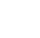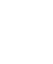Objectives
After completing this lesson, you will be able to:

After completing this lesson, you will be able to:

• Calculate data in stories
• Configure cross calculations and table formulas

## Calculated Measures

### Calculation Editor

The calculation editor allows you to create calculations for use in a chart or table. In a table, you apply calculations either to the account or to the cross calculations dimension. For each type of calculation, a new calculated or restricted member is created for the dimension that you used to create it. You can also use dimension attributes as part of a calculation.

You can display the calculation in a table by adding the account or cross calculations dimension to the table, or by selecting it in the filter applied to the dimension.

• Calculated Measures

Perform a calculation on one or more members of either the account dimension or the cross calculations dimension. A new calculated member of the selected dimension is created as a result.

• Restricted Measures

Restrict the data from a member of either the account dimension or the cross calculations dimension, so that it excludes certain members of one or more dimensions. For the date dimension, you can pick dynamic values, such as year-to-date or previous quarter. A new restricted member of the selected dimension is created as a result.

• Difference From

Find the difference in an account’s value between two dates. A new calculated account member is created as a result.

• Currency Conversion

For models with currency conversion enabled, add a currency conversion using cross calculations.

In a chart, calculations based on the account dimension can be added as new measures. The figure below shows the supported calculation types.Note
With the introduction of the new model, there are even more options when it comes to calculations:
• You can create calculated measures in the model in cases where they need to be shared across stories.
• In stories, calculation can be created on both the account dimension as well as measures.

### How to Create a Calculated Measure

Create a calculated measure in the Builder pane. When setting up your calculation, you apply the typical formula functions, conditions, and operators to the data contained in your model. Calculated measures allow you to perform mathematical and Boolean operations on your data.

Watch this video to learn how to create calculated measures.

You can add preset functions, conditions, and operators by selecting options in the Formula Functions list. You can use IF conditional functions, and you can display a list of possible formulas for the function by pressing Ctrl + Space Bar.Note
BW/Universe live models do not support dimension type calculations and there are only three options when adding measure-based calculations in the story:
• Calculated Measure

• Restricted Measure

• Aggregation

(Live models based on HANA calculation views have all of the options.)

## Calculated Dimensions

You can create calculation on dimensions for manipulate non-numeric data; for example, to concatenate dimensions together, group dimensions, etc.

Watch this video to learn how to create calculated dimensions .

When you are working with a high number of dimension members, it may be useful to group them based on a certain measure. For example, in a sales workflow you want to group sales managers based on how much revenue they are bringing in. Measure-based Calculated Dimensions allow you to easily create a new calculated dimension and define the criteria for the dimension members.

Moreover, as your data gets updated, the Measure-based Calculated Dimension members will be updated accordingly.

## Create Story Calculations

In this exercise, you will perform the following tasks:

• Create the GM % of revenue measure
• Create a restricted measure
• Create a YTD measure
• Add a Difference From measure
• Use an aggregation calculation
• Add a calculated measure with a slider bar
• Create a measure-based dimension

## Cross Calculations

Cross calculations are necessary when you have a dimension in both the Columns and the Rows of a table. For example, in the figure Dimensions in Rows and Columns the Version dimension is in the Columns and the Product dimension is in the Rows. In addition, a filter is defined for Version to restrict the view to only the Actual and Forecast versions for Gross Sales.Attempting to create a standard Calculated Measure that calculates the difference between the Actual and Forecast Gross Sales is not possible. While two restricted measure can be created: one for Actual Gross Sales and one for Forecast Gross Sales, attempting to display the Calculated measure does not produce the intended result, as seen in figure Incorrect Variance ResultsBecause Version must remain in the table in order to specify what Gross Sales we are working with, the calculated measure results for the variance is also displaying for both versions.

The work-around to this situation is to create a Cross-Calculation, so named because it allows you to calculate across multiple members of a dimension.Note
To display the selected month in the column headers, a text widget can be used that dynamically reads the filter value.

Watch this video to learn how to create a cross calculation.

To use cross calculations for currency translation, the model must have currency conversion enabled.Note
If a rolling forecast layout is needed, it is recommended to use the Forecast Layout crosstab rather than cross calculations.

## Table Formulas

You can use table formulas to perform on-the-fly, excel-like calculations. The figure below illustrates an example where you calculate percentage of sales. You can use a table formula where cell D5 is locked via \$D\$5.Note
The row number & column letters are displayed in the table but they can nevertheless be used in table formulas.

## Create Cross Calculations

You need a plan/actual/variance set of columns for all accounts.